## Neal Grantham

[email protected]

# Fill the region between two lines in ggplot2

Update (2022-05-09): This blog post presents the “Unbraided Ribbon Problem” in ggplot2 and my first attempt at a solution to it. It received a lot of positive feedback on Twitter — the “Unbraided Ribbon Problem” is more common than I thought — and a handful of readers asked if I would consider making this work into an R package. In doing so I discovered that the `ribbonize()` function at the end of this post does not work in all cases, so I got to work on a second attempt at a solution. I think I’ve finally solved it! My new package is called ggbraid and you can read more about it at nsgrantham.github.io/ggbraid. Happy braiding!

Last week I searched #ggplot on Twitter looking for a challenge and I found the following tweet:

“This shouldn’t be hard,” I thought. Wrong!

Hugh eventually solved the problem with spatial R packages `wk` and `sf`, and shared his code in this GitHub gist.

In this post I present an alternate solution using `geom_ribbon()` and a custom function I’ve written called `ribbonize()`.

## The Case of the Missing Regions

I’ll use the same fake data that Hugh used in his tweet, but I’ve renamed the variables.

``````library(tidyverse)  # 1.3.1

df <- tibble(
x = c(1:6, 1:6),
y = c(1, 5, 6, 4, 1, 1, 1, 4, 5, 4, 2, 2),
f = c(rep("a", 6), rep("b", 6))
)

df
``````
``````## # A tibble: 12 × 3
##        x     y f
##    <int> <dbl> <chr>
##  1     1     1 a
##  2     2     5 a
##  3     3     6 a
##  4     4     4 a
##  5     5     1 a
##  6     6     1 a
##  7     1     1 b
##  8     2     4 b
##  9     3     5 b
## 10     4     4 b
## 11     5     2 b
## 12     6     2 b
``````

Let’s plot `df` with `ggplot()`. Map `x` to `x`, `y` to `y` and `f` to `linetype`. Apply the line geometry with `geom_line()`.

``````ggplot(df) +
geom_line(aes(x, y, linetype = f)) +
guides(linetype = "none") +
labs(x = NULL, y = NULL)
``````To fill the regions between these two lines with `geom_ribbon()`, we’ll need to define a data frame with variables that we can map to the following aesthetics: `x`, `ymax`, `ymin`, and `fill`.

In this case, it’s easiest to pivot `df` wider so that the levels in `f` become two different columns with values from `y`. Then we use pairwise max and min, `pmax` and `pmin`, to find `ymax` and `ymin` respectively. We define the `fill` variable to be `a >= b` so that it takes values `TRUE` and `FALSE`.

``````bounds <- df %>%
pivot_wider(names_from = f, values_from = y) %>%
mutate(
ymax = pmax(a, b),
ymin = pmin(a, b),
fill = a >= b
)

bounds
``````
``````## # A tibble: 6 × 6
##       x     a     b  ymax  ymin fill
##   <int> <dbl> <dbl> <dbl> <dbl> <lgl>
## 1     1     1     1     1     1 TRUE
## 2     2     5     4     5     4 TRUE
## 3     3     6     5     6     5 TRUE
## 4     4     4     4     4     4 TRUE
## 5     5     1     2     2     1 FALSE
## 6     6     1     2     2     1 FALSE
``````

Take the previous plot and add a new layer to it by applying `geom_ribbon()` to `bounds` and mapping `x` to `x`, `ymin` to `ymin`, `ymax` to `ymax`, and `fill` to `fill`. Also, include some transparency in the colors with `alpha = 0.4`.

``````ggplot(df) +
geom_line(aes(x, y, linetype = f)) +
geom_ribbon(data = bounds, aes(x, ymin = ymin, ymax = ymax, fill = fill), alpha = 0.4) +
guides(linetype = "none", fill = "none") +
labs(x = NULL, y = NULL)
``````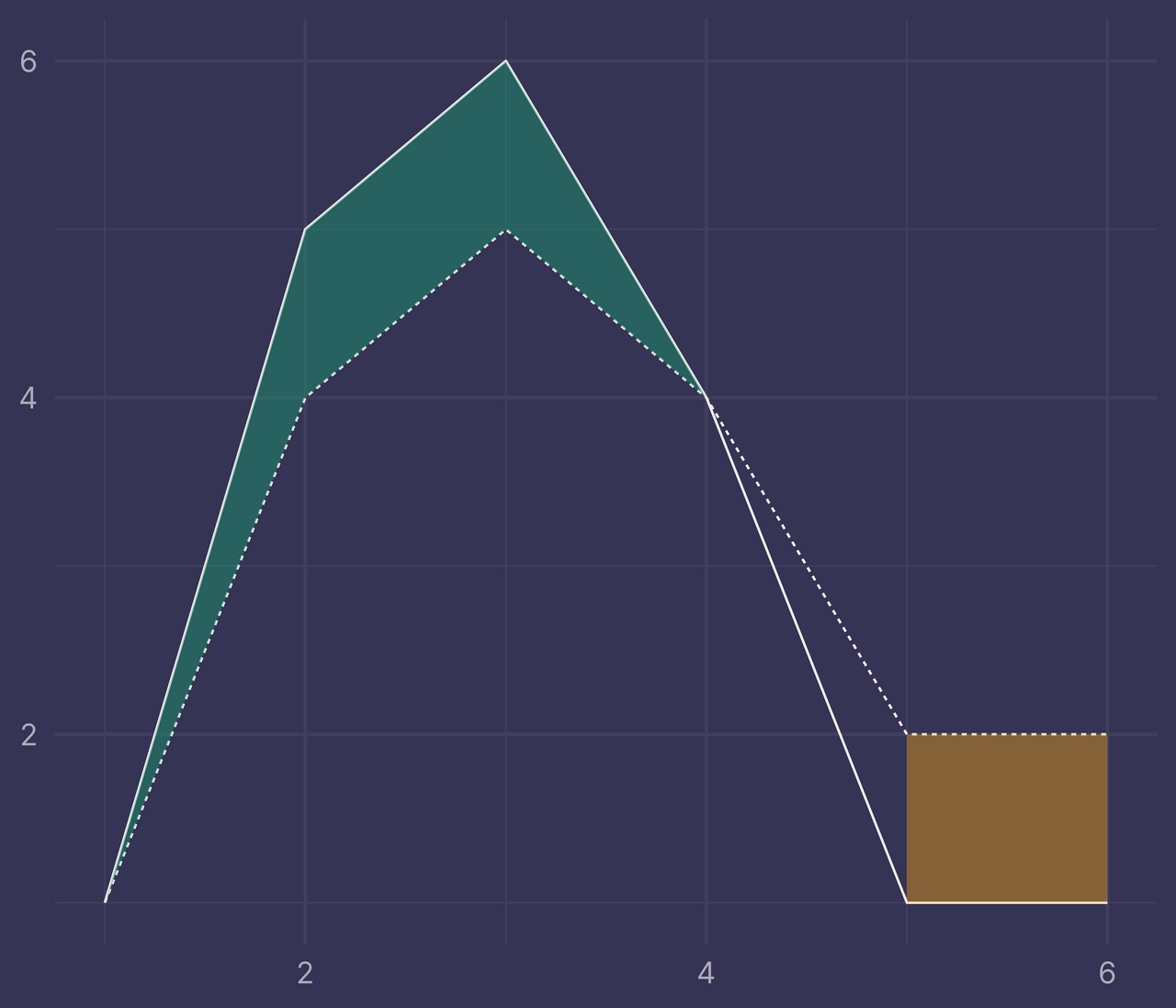Ugh. That’s not right. What did we do wrong?

I tracked down a GitHub issue from 2016 that describes the problem we’re facing. There is some discussion on what is causing this. “I don’t think this is a ggplot2 bug, I think this is expected behavior,” comments @jonocarroll. @hadley weighs in, acknowledging the behavior and suggesting that “this is better fixed outside of ggplot2.”

As far as I can tell, this problem hasn’t been fixed inside or outside of ggplot2 in the 5 years since this GitHub issue was opened.

## It’s Not a Bug, It’s a Feature

The first question to ask is: why is this happening?

Let’s take another look at `bounds`.

``````bounds
``````
``````## # A tibble: 6 × 6
##       x     a     b  ymax  ymin fill
##   <int> <dbl> <dbl> <dbl> <dbl> <lgl>
## 1     1     1     1     1     1 TRUE
## 2     2     5     4     5     4 TRUE
## 3     3     6     5     6     5 TRUE
## 4     4     4     4     4     4 TRUE
## 5     5     1     2     2     1 FALSE
## 6     6     1     2     2     1 FALSE
``````

When `x` is `1`, `2`, `3`, or `4`, `fill` is `TRUE` and `geom_ribbon()` fills the region between the intersections at `x = 1` and `x = 4`.

When `x` is `5` or `6`, `fill` is `FALSE` and `geom_ribbon()` fills the region between these two values (with a different color than when `fill` is `TRUE`).

But what about the region between `4` and `5`? There’s nothing in `bounds` that would tell `geom_ribbon()` to fill this region — as far as it knows, `fill = TRUE` between `x = 1` and `x = 4` and `fill = FALSE` between `x = 5` and `x = 6`.

We must append a new row to `bounds` so `geom_ribbon()` knows that `fill = FALSE` begins at `x = 4`, not `x = 5`.

``````bounds2 <- bind_rows(
bounds,
tibble(x = 4, a = 4, b = 4, ymax = 4, ymin = 4, fill = FALSE)
) %>%
arrange(x)

bounds2
``````
``````## # A tibble: 7 × 6
##       x     a     b  ymax  ymin fill
##   <dbl> <dbl> <dbl> <dbl> <dbl> <lgl>
## 1     1     1     1     1     1 TRUE
## 2     2     5     4     5     4 TRUE
## 3     3     6     5     6     5 TRUE
## 4     4     4     4     4     4 TRUE
## 5     4     4     4     4     4 FALSE
## 6     5     1     2     2     1 FALSE
## 7     6     1     2     2     1 FALSE
``````
``````ggplot(df) +
geom_line(aes(x, y, linetype = f)) +
geom_ribbon(data = bounds2, aes(x, ymin = ymin, ymax = ymax, fill = fill), alpha = 0.4) +
guides(linetype = "none", fill = "none") +
labs(x = NULL, y = NULL)
``````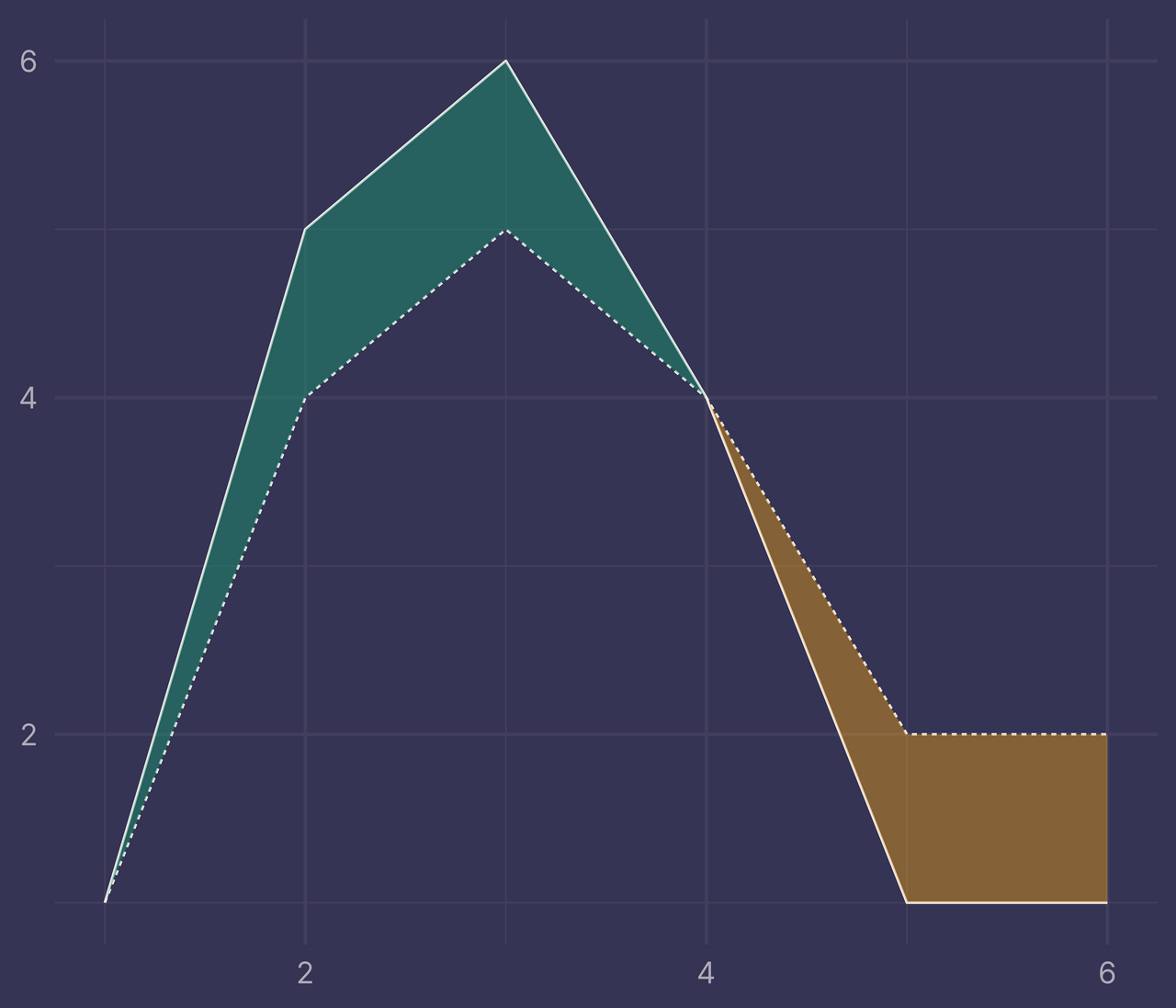Success!

So the problem is not caused by a bug in ggplot2.

The real problem is that we were not passing the right data to `geom_ribbon()` so it was unable to fill the regions correctly.

## Intervals and Intersections

Now the next question to ask is: how do I get the right data?

There are many ways to approach this. Of course, we can stick with the manual approach above, adding new rows to `bounds` as they’re required. But ideally we’d find a programmatic way to get the right data every time.

To do so, it’s helpful to separate `bounds` into two different data frames: `intervals` and `intersections`.

Filter `bounds` on `ymax > ymin` and assign it to `intervals`.

``````intervals <- bounds %>%
filter(ymax > ymin)

intervals
``````
``````## # A tibble: 4 × 6
##       x     a     b  ymax  ymin fill
##   <int> <dbl> <dbl> <dbl> <dbl> <lgl>
## 1     2     5     4     5     4 TRUE
## 2     3     6     5     6     5 TRUE
## 3     5     1     2     2     1 FALSE
## 4     6     1     2     2     1 FALSE
``````

Leave `intervals` alone (since it already behaves correctly when passed to `geom_ribbon()`).

Next, filter `bounds` on `ymax == ymin` and assign it to `intersections`.

``````intersections <- bounds %>%
filter(ymax == ymin)

intersections
``````
``````## # A tibble: 2 × 6
##       x     a     b  ymax  ymin fill
##   <int> <dbl> <dbl> <dbl> <dbl> <lgl>
## 1     1     1     1     1     1 TRUE
## 2     4     4     4     4     4 TRUE
``````

`intersections` requires some additional work to get right.

We have an intersection at `x = 1` where `fill = TRUE` begins.

We also have an intersection at `x = 4` where `fill = TRUE` ends and `fill = FALSE` begins. However, only `fill = TRUE` is captured in `intersections` so we must transform `intersections` to include a new row.

To do so, we will use `arrange()` to sort the rows of `bounds` by `x` and define two new variables, `lag_fill` and `lead_fill`, which record the previous and next `fill` values respectively.

``````intersections <- bounds %>%
arrange(x) %>%
filter(ymax == ymin)

intersections
``````
``````## # A tibble: 2 × 8
##       x     a     b  ymax  ymin fill  lag_fill lead_fill
##   <int> <dbl> <dbl> <dbl> <dbl> <lgl> <lgl>    <lgl>
## 1     1     1     1     1     1 TRUE  NA       TRUE
## 2     4     4     4     4     4 TRUE  TRUE     FALSE
``````

When `x = 1`, `lag_fill` is `NA` because there is no previous row and `lead_fill` is `TRUE` since `fill` is `TRUE` at the next row `x = 2`.

When `x = 4`, `lag_fill` is `TRUE` because the previous row has `x = 3` and `fill = TRUE` and `lead_fill` is `FALSE` because the next row has `x = 5` and `fill = FALSE`.

Now, we can pivot longer and replace `fill` with the values of `lag_fill` and `lead_fill`. Further, remove any rows where `fill` is `NA` and remove duplicate rows, should they exist, with `distinct()`.

``````intersections <- bounds %>%
arrange(x) %>%
filter(ymax == ymin) %>%
select(-fill) %>%
pivot_longer(lag_fill:lead_fill, names_to = NULL, values_to = "fill") %>%
filter(!is.na(fill)) %>%
distinct()

intersections
``````
``````## # A tibble: 3 × 6
##       x     a     b  ymax  ymin fill
##   <int> <dbl> <dbl> <dbl> <dbl> <lgl>
## 1     1     1     1     1     1 TRUE
## 2     4     4     4     4     4 TRUE
## 3     4     4     4     4     4 FALSE
``````

Bind `intervals` and `intersections` to create a new data frame, `ribbons`, and pass this to `geom_ribbon()` instead of `bounds`.

``````ribbons <- bind_rows(
intervals,
intersections
)

ggplot(df) +
geom_line(aes(x, y, linetype = f)) +
geom_ribbon(data = ribbons, aes(x, ymin = ymin, ymax = ymax, fill = fill), alpha = 0.4) +
guides(linetype = "none", fill = "none") +
labs(x = NULL, y = NULL)
``````We did it!

Alright, so we’re done?

Not so fast.

## Other Intersections

Suppose we add a few new rows to the original data.

``````df <- tibble(
x = c(1:8, 1:8),
y = c(1, 5, 6, 4, 1, 1, 3, 2, 1, 4, 5, 4, 2, 2, 2, 2),
f = c(rep("a", 8), rep("b", 8))
)

ggplot(df, aes(x, y, linetype = f)) +
geom_line() +
guides(linetype = "none") +
labs(x = NULL, y = NULL)
``````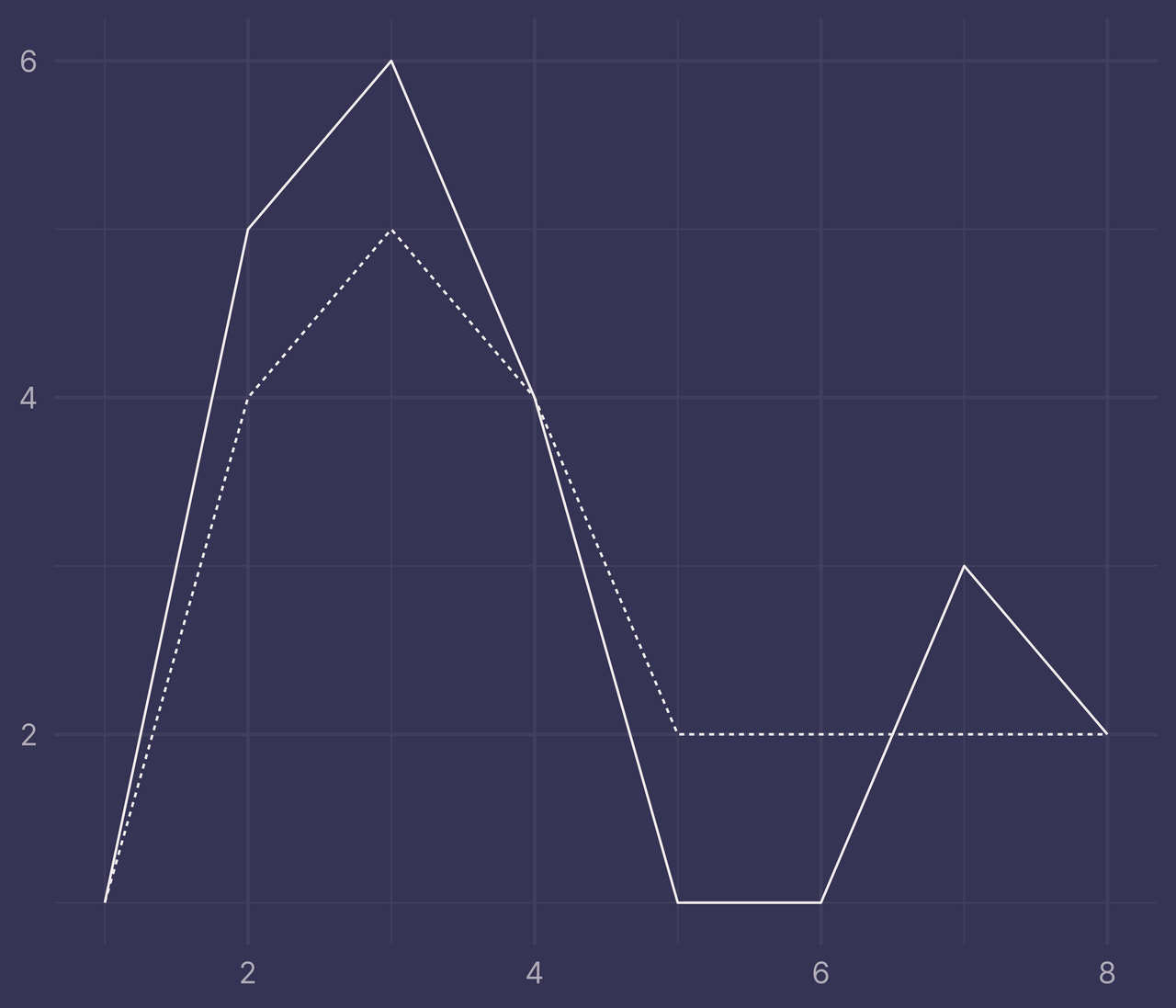Now repeat the same steps as before with `intervals` and `intersections` (but this time we’ll remove the `a` and `b` variables when they’re no longer needed).

``````bounds <- df %>%
pivot_wider(names_from = f, values_from = y) %>%
mutate(
ymax = pmax(a, b),
ymin = pmin(a, b),
fill = a > b
)

intervals <- bounds %>%
filter(ymax > ymin) %>%
select(-a, -b)

intersections <- bounds %>%
filter(ymax == ymin) %>%
select(-a, -b, -fill) %>%
pivot_longer(lag_fill:lead_fill, names_to = NULL, values_to = "fill") %>%
filter(!is.na(fill)) %>%
distinct()

ribbons <- bind_rows(
intervals,
intersections
) %>%
arrange(x)

ribbons
``````
``````## # A tibble: 9 × 4
##       x  ymax  ymin fill
##   <int> <dbl> <dbl> <lgl>
## 1     1     1     1 TRUE
## 2     2     5     4 TRUE
## 3     3     6     5 TRUE
## 4     4     4     4 TRUE
## 5     4     4     4 FALSE
## 6     5     2     1 FALSE
## 7     6     2     1 FALSE
## 8     7     3     2 TRUE
## 9     8     2     2 TRUE
``````
``````ggplot(df) +
geom_line(aes(x, y, linetype = f)) +
geom_ribbon(data = ribbons, aes(x, ymin = ymin, ymax = ymax, fill = fill), alpha = 0.4) +
guides(linetype = "none", fill = "none") +
labs(x = NULL, y = NULL)
``````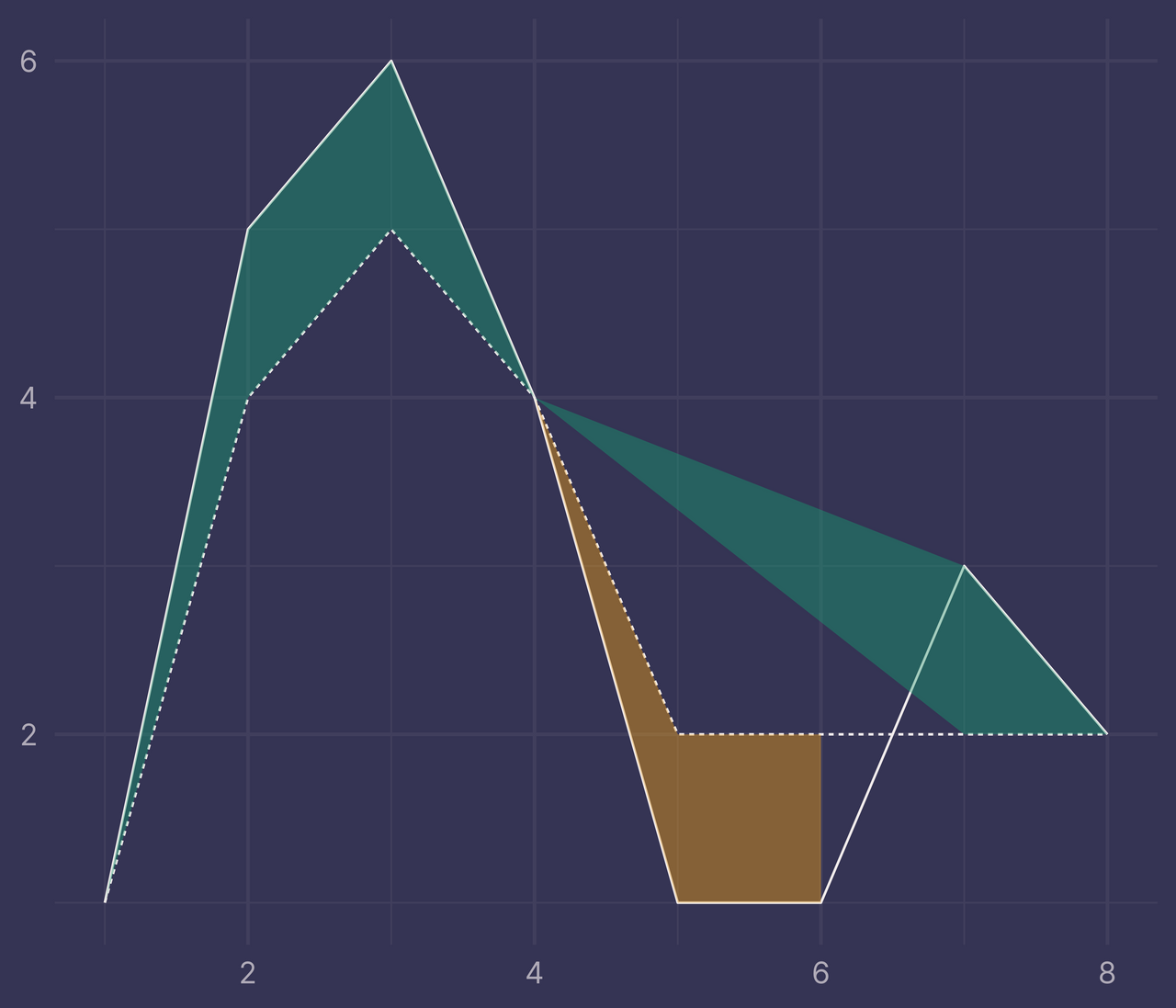Bleh. It looks kinda cool, but it’s not at all what we want.

So, why is this happening?

There’s a new intersection that occurs at `x = 6.5` but we have no such row in `ribbons`, only `x = 6` and `x = 7`.

To accommodate this new intersection, we’ll need to calculate its position and add it as a new row to `ribbons`.

Thankfully there’s a formula we can use to find the position of an intersection point given two points on each line.

To make things easier to follow, I’ll use similar variable names as in the formula. The following steps may look a little complicated, but it’s not necessary to follow along completely. Just know that we are applying the formula in a tidy way to find any intersections that occur between two `x`.

``````other_intersections <- bounds %>%
transmute(
x1 = x,       y1 = a,
x3 = x,       y3 = b,
) %>%
filter(((y1 > y3) & (y2 < y4)) | ((y1 < y3) & (y2 > y4))) %>%  # only rows where an intersection occurs between two x
mutate(
d = (x1 - x2) * (y3 - y4) - (y1 - y2) * (x3 - x4),  # denominator
u = x1 * y2 - y1 * x2,
v = x3 * y4 - y3 * x4,
x = (u * (x3 - x4) - v * (x1 - x2)) / d,
y = (u * (y3 - y4) - v * (y1 - y2)) / d
) %>%
select(x, ymax = y, ymin = y)

other_intersections
``````
``````## # A tibble: 1 × 3
##       x  ymax  ymin
##   <dbl> <dbl> <dbl>
## 1   6.5     2     2
``````

Now points in `other_intersections` will always mark transitions from `fill = TRUE` to `fill = FALSE` or the other way around, so we’ll bind two versions of `other_intersections` to `intervals` and `intersections`, one where `fill = TRUE` and another where `fill = FALSE`.

``````ribbons <- bind_rows(
intervals,
intersections,
mutate(other_intersections, fill = TRUE),
mutate(other_intersections, fill = FALSE)
) %>%
arrange(x)

ribbons
``````
``````## # A tibble: 11 × 4
##        x  ymax  ymin fill
##    <dbl> <dbl> <dbl> <lgl>
##  1   1       1     1 TRUE
##  2   2       5     4 TRUE
##  3   3       6     5 TRUE
##  4   4       4     4 TRUE
##  5   4       4     4 FALSE
##  6   5       2     1 FALSE
##  7   6       2     1 FALSE
##  8   6.5     2     2 TRUE
##  9   6.5     2     2 FALSE
## 10   7       3     2 TRUE
## 11   8       2     2 TRUE
``````

Putting this all together, we plot again and…

``````ggplot(df) +
geom_line(aes(x, y, linetype = f)) +
geom_ribbon(data = ribbons, aes(x, ymin = ymin, ymax = ymax, fill = fill), alpha = 0.4) +
guides(linetype = "none", fill = "none") +
labs(x = NULL, y = NULL)
``````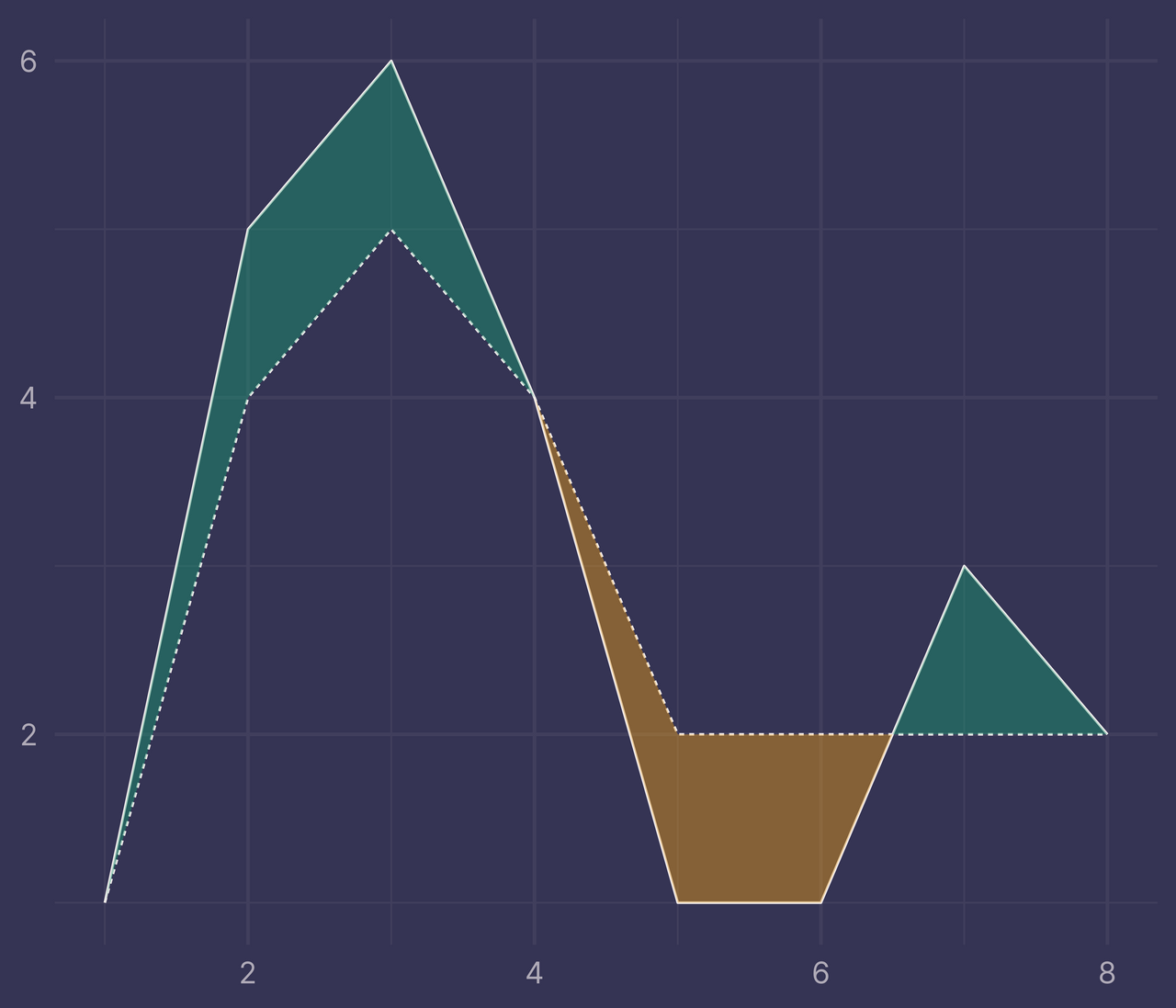Voila!

## Introducing `ribbonize()`

If you’d like to apply this to your own situation, I’ve written a function that reproduces all the same steps in this post.

I’ve called the function `ribbonize()`. Feel free to use it however you’d like — for the British English spellers, you have my permission to rename it to `ribbonise()`. :)

``````ribbonize <- function(.data, .x, .y, .f) {
# Calculate the ribbons required for geom_ribbon().
#
# Usage:
# df <- tibble(
#   x = c(1:8, 1:8),
#   y = c(1, 5, 6, 4, 1, 1, 3, 2, 1, 4, 5, 4, 2, 2, 2, 2),
#   f = c(rep("a", 8), rep("b", 8))
# )
#
# ribbons <- ribbonize(df, x, y, f)
#
# ggplot(df) +
#   geom_line(aes(x, y, linetype = f)) +
#   geom_ribbon(data = ribbons, aes(x, ymin = ymin, ymax = ymax, fill = fill))

# Check there are only 2 level in .f
levels <- .data %>%
pull({{ .f }}) %>%
unique()

stopifnot(length(levels) == 2)

# Check that there is exactly 1 observation per level in .f at every .x
level_counts_by_x <- .data %>%
filter(!is.na({{ .y }})) %>%
group_by({{ .x }}) %>%
count() %>%
pull(n)

stopifnot(all(level_counts_by_x == 2))

bounds <- .data %>%
mutate({{ .f }} := recode({{ .f }}, a = levels, b = levels)) %>%
pivot_wider(names_from = {{ .f }}, values_from = {{ .y }}) %>%
mutate(
ymax = pmax(a, b),
ymin = pmin(a, b),
fill = a >= b
)

intervals <- bounds %>%
filter(ymax > ymin) %>%
select(-a, -b)

intersections <- bounds %>%
filter(ymax == ymin) %>%
select(-a, -b, -fill) %>%
pivot_longer(lag_fill:lead_fill, names_to = NULL, values_to = "fill") %>%
filter(!is.na(fill)) %>%
distinct()

other_intersections <- bounds %>%
transmute(
x1 = {{ .x }},       y1 = a,
x3 = {{ .x }},       y3 = b,
) %>%
filter(((y1 > y3) & (y2 < y4)) | ((y1 < y3) & (y2 > y4))) %>%
mutate(
d = (x1 - x2) * (y3 - y4) - (y1 - y2) * (x3 - x4),
u = x1 * y2 - y1 * x2,
v = x3 * y4 - y3 * x4,
x = (u * (x3 - x4) - v * (x1 - x2)) / d,
y = (u * (y3 - y4) - v * (y1 - y2)) / d
) %>%
select(x, ymax = y, ymin = y)

bind_rows(
intervals,
intersections,
mutate(other_intersections, fill = TRUE),
mutate(other_intersections, fill = FALSE)
) %>%
arrange({{ .x }})
}
``````
``````ribbons <- ribbonize(df, x, y, f)

ggplot(df) +
geom_line(aes(x, y, linetype = f)) +
geom_ribbon(data = ribbons, aes(x, ymin = ymin, ymax = ymax, fill = fill), alpha = 0.4) +
guides(linetype = "none", fill = "none") +
labs(x = NULL, y = NULL)
``````And for a sanity check, let’s try it out on a randomly generated dataset.

``````set.seed(42)  # for reproducibility

df <- tibble(
x = c(1:20, 1:20),
y = c(rnorm(20), rnorm(20, mean = 0.5)),
f = c(rep("a", 20), rep("b", 20))
)

ggplot(df, aes(x, y, linetype = f)) +
geom_line() +
guides(linetype = "none") +
labs(x = NULL, y = NULL)
``````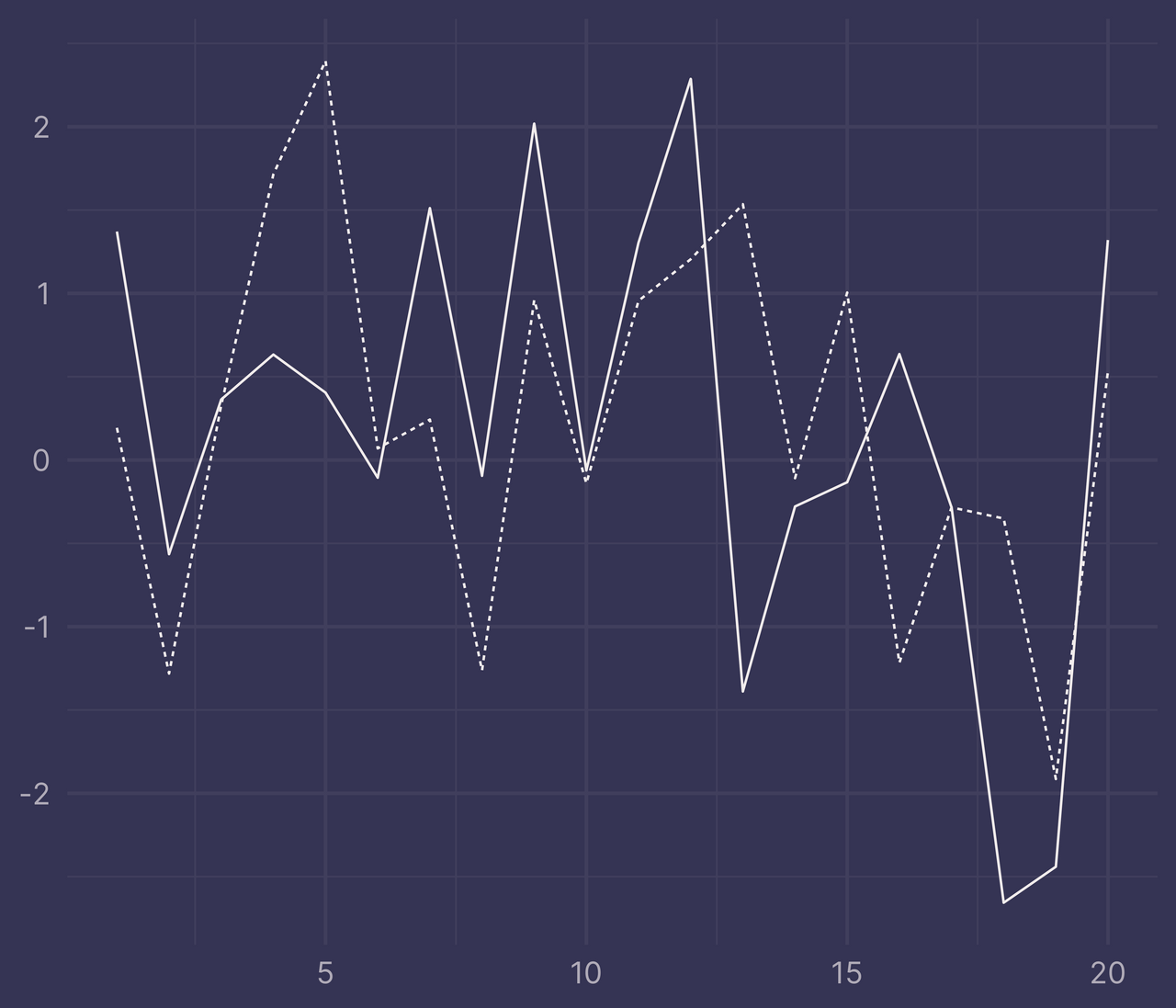``````ribbons <- ribbonize(df, x, y, f)

ggplot(df) +
geom_line(aes(x, y, linetype = f)) +
geom_ribbon(data = ribbons, aes(x, ymin = ymin, ymax = ymax, fill = fill), alpha = 0.4) +
guides(linetype = "none", fill = "none") +
labs(x = NULL, y = NULL)
``````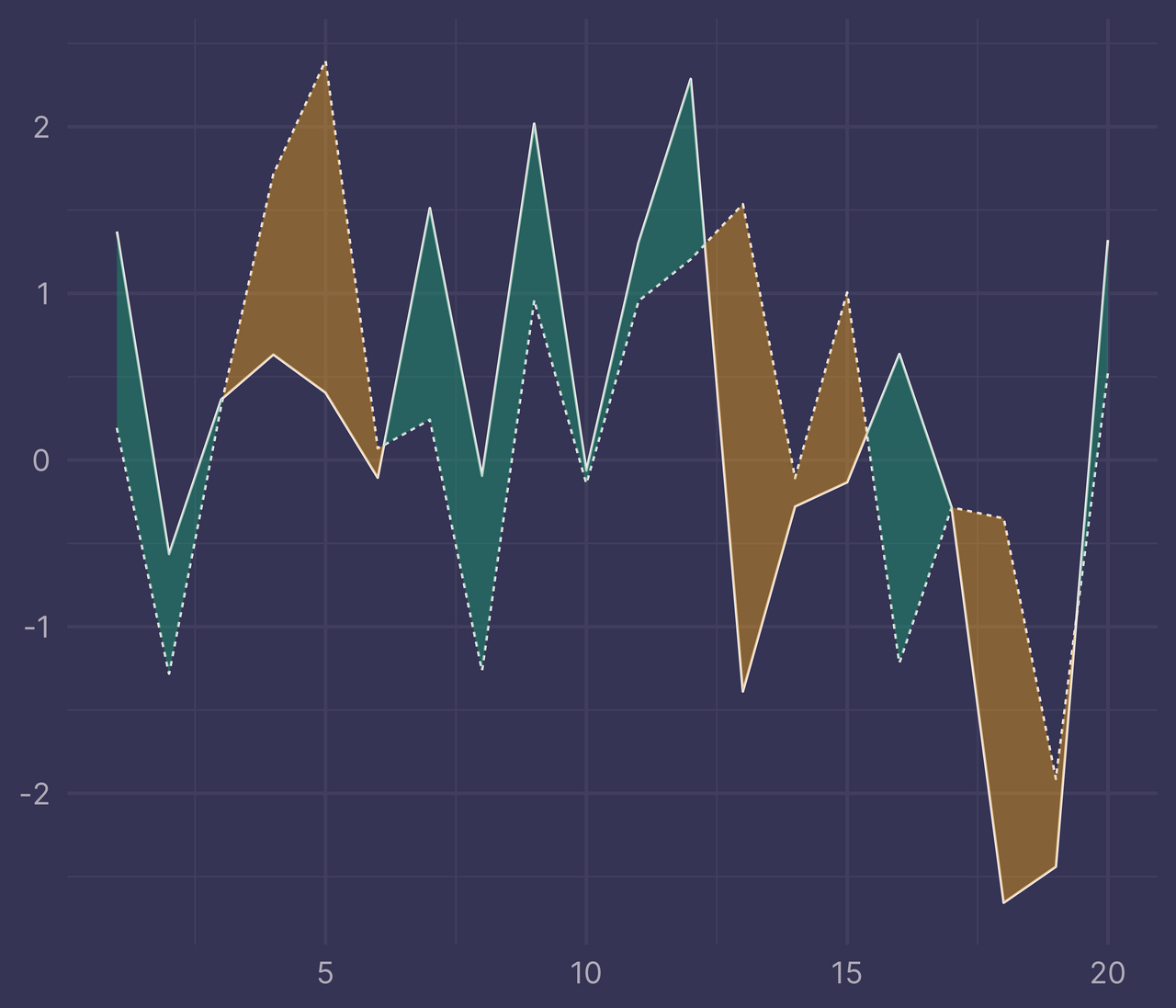Perfecto!

## When doesn’t this work?

`ribbonize()` has a couple requirements to work properly.

First, it only works for data with exactly two factor levels. Any more, or any less, and it will throw an error.

``````df <- tibble(
x = c(1:6, 1:6, 1:6),
y = c(1, 5, 6, 4, 1, 1, 1, 4, 5, 4, 2, 2, 5, 2, 4, 2, 1, 3),
f = c(rep("a", 6), rep("b", 6), rep("c", 6))
)

ggplot(df) +
geom_line(aes(x, y, linetype = f)) +
guides(linetype = "none") +
labs(x = NULL, y = NULL)
``````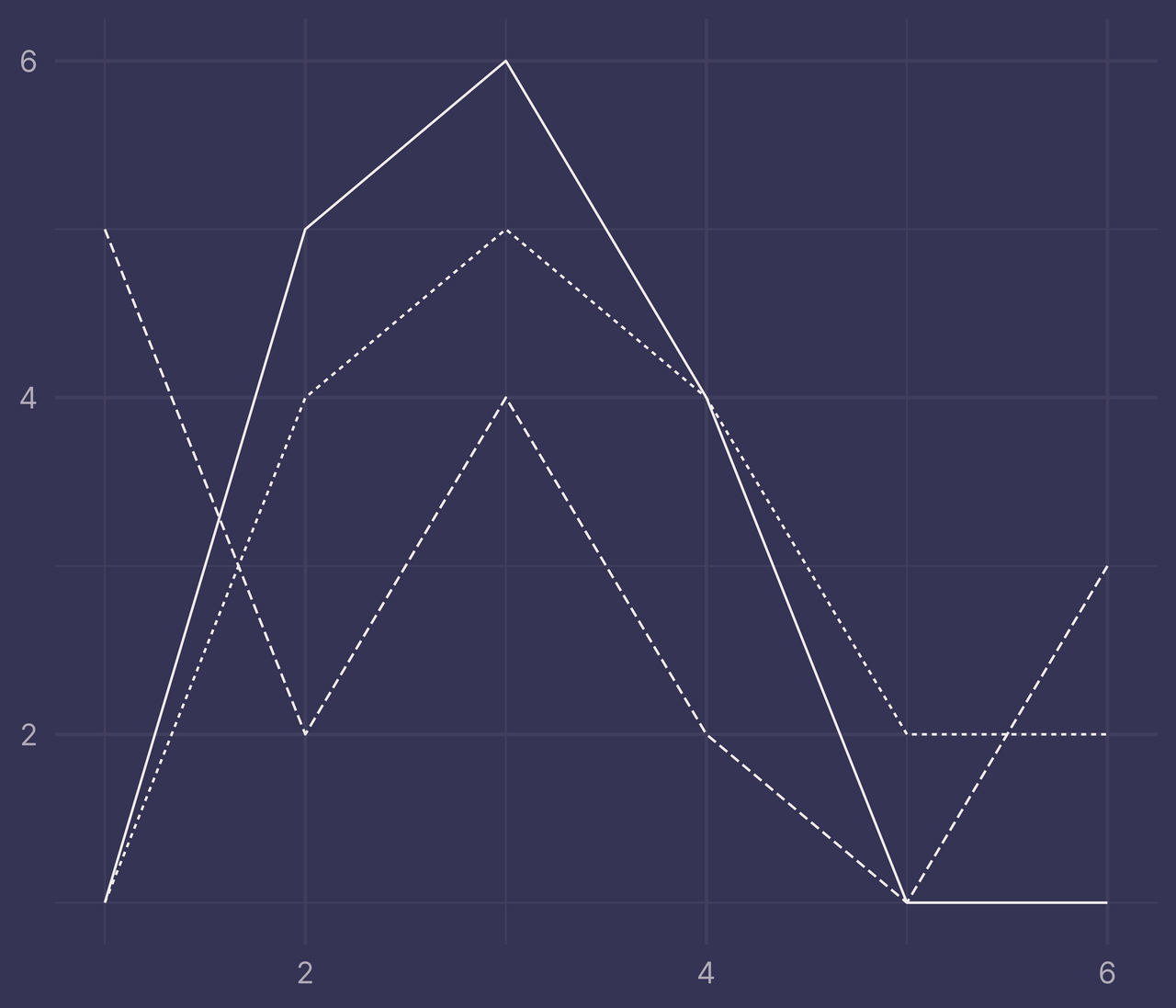``````ribbons <- ribbonize(df, x, y, f)
``````
``````## Error in ribbonize(df, x, y, f): length(levels) == 2 is not TRUE
``````

Second, for every `x` in `df` there must be a corresponding value `y` for each level in `f`, otherwise `ribbonize()` will throw an error.

``````df <- tibble(
x = c(1:6, 1:6),
y = c(1, 5, 6, NA, 1, 1, 1, 4, 5, 4, NA, 2),
f = c(rep("a", 6), rep("b", 6))
)

df
``````
``````## # A tibble: 12 × 3
##        x     y f
##    <int> <dbl> <chr>
##  1     1     1 a
##  2     2     5 a
##  3     3     6 a
##  4     4    NA a
##  5     5     1 a
##  6     6     1 a
##  7     1     1 b
##  8     2     4 b
##  9     3     5 b
## 10     4     4 b
## 11     5    NA b
## 12     6     2 b
``````

If we filter out the rows with `NA` and pass it to `ggplot()`, we can still plot it correctly (I added points with `geom_point()` so it’s easier to see where we’re missing data).

``````df %>%
filter(!is.na(y)) %>%
ggplot() +
geom_line(aes(x, y, linetype = f)) +
geom_point(aes(x, y)) +
guides(linetype = "none") +
labs(x = NULL, y = NULL)
``````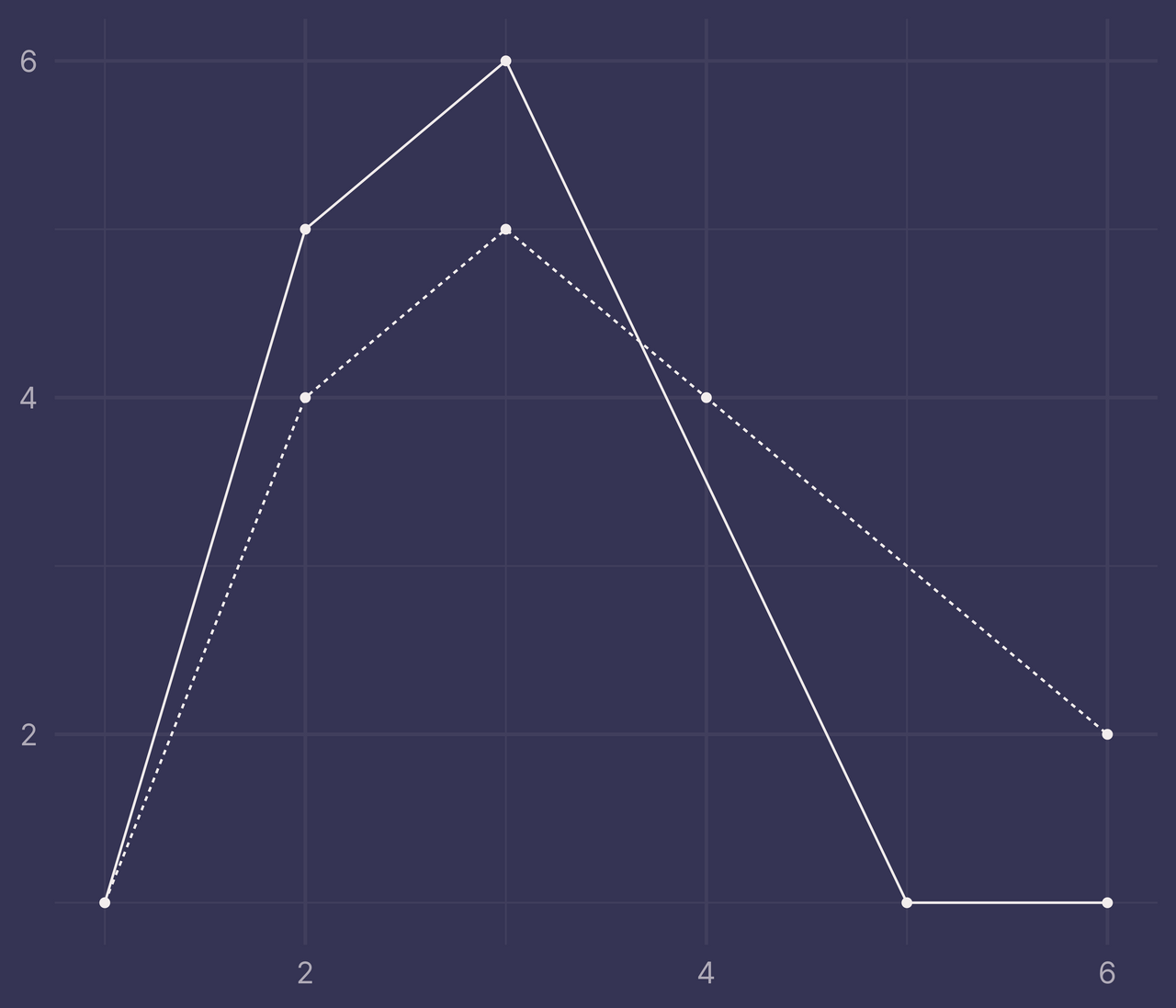But if this same data is passed to `ribbonize()` it throws an error.

``````ribbons <- ribbonize(df, x, y, f)
``````
``````## Error in ribbonize(df, x, y, f): all(level_counts_by_x == 2) is not TRUE
``````

The function can be modified to accommodate data like this, but I’ll leave that work for a future blog post.

## Wrapping Up

If you use `ribbonize()` in your own work and run into any issues, reach out to me on Twitter.

Happy ribbonizing! 🎀

September 29, 2021  @nsgrantham